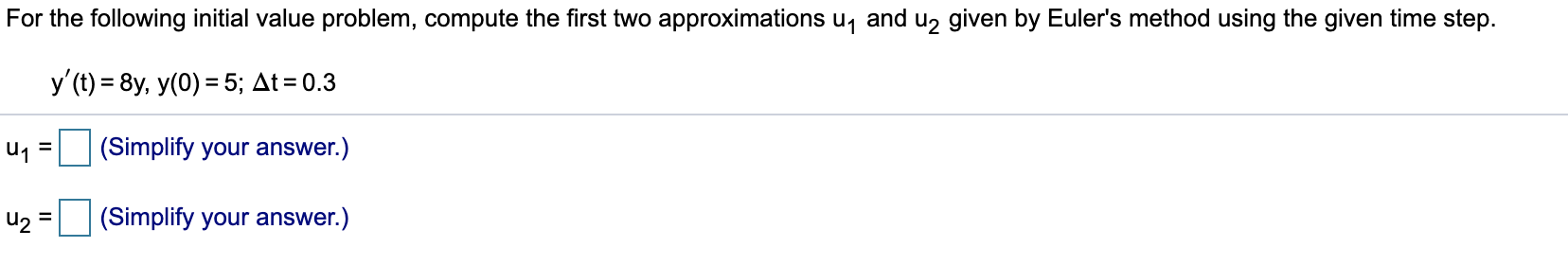# For the following initial value problem, compute the first two approximations u, and u, given by Euler's method using the given time step. y'(t) = 8y, y(0) = 5; At = 0.3 u1 = (Simplify your answer.) (Simplify your answer.) %3D

Questionhelp_outlineImage TranscriptioncloseFor the following initial value problem, compute the first two approximations u, and u, given by Euler's method using the given time step. y'(t) = 8y, y(0) = 5; At = 0.3 u1 = (Simplify your answer.) (Simplify your answer.) %3D fullscreen

1 Rating

### Want to see this answer and more?

Experts are waiting 24/7 to provide step-by-step solutions in as fast as 30 minutes!*

*Response times may vary by subject and question complexity. Median response time is 34 minutes for paid subscribers and may be longer for promotional offers.
Tagged in
Math
Calculus

### Other